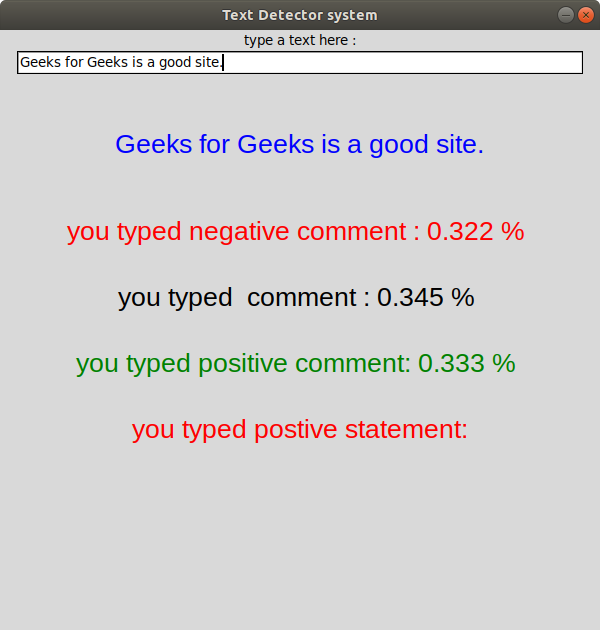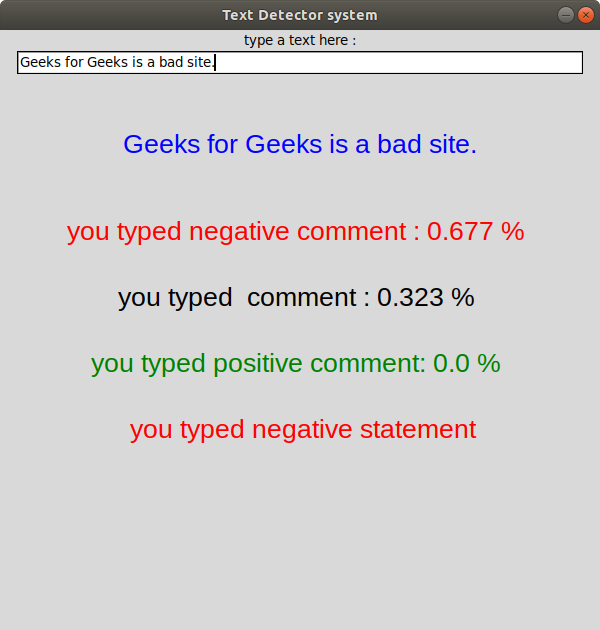# Text detection using Python

• Last Updated : 14 Sep, 2021

Python language is widely used for modern machine learning and data analysis. One can detect an image, speech, can even detect an object through Python. For now, we will detect whether the text from the user gives a positive feeling or negative feeling by classifying the text as positive, negative, or neutral. In the code, Vader sentiment analysis and Tkinter are used. Tkinter is a standard GUI library for creating the GUI application.

Required Installations in Anaconda:

• tkinter: This module is used for creating a simple GUI application. This module generally comes pre-installed with Python but to install it externally type the below command in the terminal.
Using conda command.
`conda install -c anaconda tk`

Linux users can also use the below command.

`sudo apt-get install python3-tk`
• nltk: This module is used for making computers understand the natural language. To install it type the below command in the terminal.
Using conda.
`conda install -c anaconda nltk`

Using pip.

`pip install nltk`
• numpy: This module is the fundamental package for scientific computing with Python. To install it type the below command in the terminal.
Using conda.
`conda install -c conda-forge numpy`

Using pip.

`pip install numpy`
• pandas: This module is used for data analysis. It provides highly optimized performance with back-end source code is purely written in C or Python. To install it type the below command in the terminal.
Using conda
`conda install -c anaconda pandas`

Using pip.

`pip install pandas`
• matplotlib: This module is an amazing visualization library in Python for 2D plots of arrays. Matplotlib is a multi-platform data visualization library built on NumPy arrays. To install it type the below command in the terminal.
Using conda.
`conda install -c conda-forge matplotlib`

Using pip.

`pip install matplotlib`

VADER (Valence Aware Dictionary and sEntiment Reasoner) is a lexicon and rule-based sentiment analysis tool that is specifically attuned to sentiments expressed in social media. VADER uses a combination of A sentiment lexicon is a list of lexical features (e.g., words) which are generally labeled according to their semantic orientation as either positive or negative. VADER not only tells about the Positivity and Negativity score but also tells us about how positive or negative a sentiment is.

Below is the implementation.

## Python3

 `import` `time``import` `pandas as pd``import` `numpy as np``import` `matplotlib.pyplot as plt``from` `tkinter ``import` `*``import` `tkinter.messagebox``from` `nltk.sentiment.vader ``import` `SentimentIntensityAnalyzer`` ` `    ` `class` `analysis_text():``    ` `    ``# Main function in program``    ``def` `center(``self``, toplevel):``        ` `        ``toplevel.update_idletasks()``        ``w ``=` `toplevel.winfo_screenwidth()``        ``h ``=` `toplevel.winfo_screenheight()``        ``size ``=` `tuple``(``int``(_) ``for` `_ ``in``                     ``toplevel.geometry().split(``'+'``)[``0``].split(``'x'``))``        ` `        ``x ``=` `w``/``2` `-` `size[``0``]``/``2``        ``y ``=` `h``/``2` `-` `size[``1``]``/``2``        ``toplevel.geometry(``"%dx%d+%d+%d"` `%` `(size ``+` `(x, y)))`` ` `    ``def` `callback(``self``):``        ``if` `tkinter.messagebox.askokcancel(``"Quit"``,``                                          ``"Do you want to leave?"``):``            ``self``.main.destroy()`` ` `    ``def` `setResult(``self``, ``type``, res):``        ` `        ``#calculated comments in vader analysis``        ``if` `(``type` `=``=` `"neg"``):``            ``self``.negativeLabel.configure(text ``=``                                         ``"you typed negative comment : "``                                         ``+` `str``(res) ``+` `" % \n"``)``        ``elif` `(``type` `=``=` `"neu"``):``            ``self``.neutralLabel.configure( text ``=``                                        ``"you typed  comment : "``                                        ``+` `str``(res) ``+` `" % \n"``)``        ``elif` `(``type` `=``=` `"pos"``):``            ``self``.positiveLabel.configure(text``                                        ``=` `"you typed positive comment: "``                                         ``+` `str``(res) ``+` `" % \n"``)``        ` ` ` `    ``def` `runAnalysis(``self``):``        ` `        ``sentences ``=` `[]``        ``sentences.append(``self``.line.get())``        ``sid ``=` `SentimentIntensityAnalyzer()``        ` `        ``for` `sentence ``in` `sentences:``            ` `            ``# print(sentence)``            ``ss ``=` `sid.polarity_scores(sentence)``            ` `            ``if` `ss[``'compound'``] >``=` `0.05` `:``                ``self``.normalLabel.configure(text ``=``                                           ``" you typed positive statement: "``)``   ` `            ``elif` `ss[``'compound'``] <``=` `-` `0.05` `:``                ``self``.normalLabel.configure(text ``=``                                           ``" you typed negative statement"``)``   ` `            ``else` `:``             ``self``.normalLabel.configure(text ``=``                                        ``" you normal typed  statement: "``)``            ``for` `k ``in` `sorted``(ss):``                ``self``.setResult(k, ss[k])``        ``print``()``        ` ` ` `    ``def` `editedText(``self``, event):``        ``self``.typedText.configure(text ``=` `self``.line.get() ``+` `event.char)``        ` ` ` `    ``def` `runByEnter(``self``, event):``        ``self``.runAnalysis()`` `  `    ``def` `__init__(``self``):``        ``# Create main window``        ``self``.main ``=` `Tk()``        ``self``.main.title(``"Text Detector system"``)``        ``self``.main.geometry(``"600x600"``)``        ``self``.main.resizable(width``=``FALSE, height``=``FALSE)``        ``self``.main.protocol(``"WM_DELETE_WINDOW"``, ``self``.callback)``        ``self``.main.focus()``        ``self``.center(``self``.main)`` ` `        ``# addition item on window``        ``self``.label1 ``=` `Label(text ``=` `"type a text here :"``)``        ``self``.label1.pack()`` ` `        ``# Add a hidden button Enter``        ``self``.line ``=` `Entry(``self``.main, width``=``70``)``        ``self``.line.pack()`` ` `        ``self``.textLabel ``=` `Label(text ``=` `"\n"``,``                               ``font``=``(``"Helvetica"``, ``15``))``        ``self``.textLabel.pack()``        ``self``.typedText ``=` `Label(text ``=` `"",``                               ``fg ``=` `"blue"``,``                               ``font``=``(``"Helvetica"``, ``20``))``        ``self``.typedText.pack()`` ` `        ``self``.line.bind(``""``,``self``.editedText)``        ``self``.line.bind(``""``,``self``.runByEnter)`` ` ` ` `        ``self``.result ``=` `Label(text ``=` `"\n"``,``                            ``font``=``(``"Helvetica"``, ``15``))``        ``self``.result.pack()``        ``self``.negativeLabel ``=` `Label(text ``=` `"",``                                   ``fg ``=` `"red"``,``                                   ``font``=``(``"Helvetica"``, ``20``))``        ``self``.negativeLabel.pack()``        ``self``.neutralLabel  ``=` `Label(text ``=` `"",``                                   ``font``=``(``"Helvetica"``, ``20``))``        ``self``.neutralLabel.pack()``        ``self``.positiveLabel ``=` `Label(text ``=` `"",``                                   ``fg ``=` `"green"``,``                                   ``font``=``(``"Helvetica"``, ``20``))``        ``self``.positiveLabel.pack()``        ``self``.normalLabel ``=``Label (text ``=``"",``                                 ``fg ``=``"red"``,``                                 ``font``=``(``"Helvetica"``, ``20``))``        ``self``.normalLabel.pack()``        ` `# Driver code``myanalysis ``=` `analysis_text()``mainloop()`

Output:My Personal Notes arrow_drop_up